# Quantitative Aptitude Quiz for Prelims Exams- SBI & IBPS 2020- 14th December

Directions (1-5): In each of these questions, two equations (I) and (II) are given. You have to solve both the equations and give answer.
(a) if x>y
(b) if x≥y
(c) if x<y
(d) if x ≤y
(e) if x = y or no relation can be established between x and y.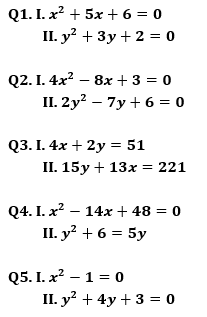Directions (6-10): In each of these questions, two equations (I) and (II) are given. You have to solve both the equations and give answer.
(a) if x>y
(b) if x≥y
(c) if x<y
(d) if x ≤y
(e) if x = y or no relation can be established between x and y.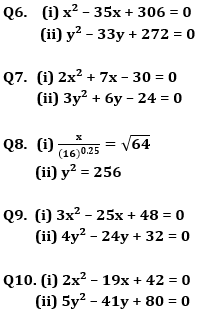Directions (11-15): In the following questions two quantities are given for each question. Compare the numeric value of both the quantities and answers accordingly.

Q11. Quantity I: Cost price and marked price of an article is Rs. 250 and Rs 375 respectively. Profit of x% is earned when discount of x% is given on it. Find selling price of article.
Quantity II: A shopkeeper sold 25 orange in Rs.45 and got 10% loss. Cost price of 150 such orange.
(a) Quantity I > Quantity II
(b) Quantity II > Quantity I
(c) Quantity I = Quantity II
(d) Quantity I ≤ Quantity II
(e) Quantity II ≤ Quantity I

Q12. Volume of a cone is 9240 cm³ and area of circular base of that cone is 1386 cm².
Quantity II: Height of cone.
(a) Quantity I ≤ Quantity II
(b) Quantity I ≥ Quantity II
(c) Quantity I = Quantity II
(d) Quantity II < Quantity I
(e) Quantity II > Quantity I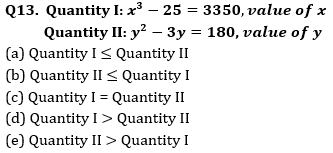Q14. Time taken by boat to cover 400 km in upstream is 32 hours more than the time taken by boat to cover 400 km in downstream and the total time taken by boat to cover 180 km in upstream is 18 hours.
Quantity I: Speed of boat in still water.
Quantity II: 30 km/hr.
(a) Quantity I > Quantity II
(b) Quantity II > Quantity I
(c) Quantity I = Quantity II
(d) Quantity I ≤ Quantity II
(e) Quantity II ≤ Quantity I

Q15. Quantity I:– A square of side 7/2 meter is bent into form of a circle. Area of circle.
Quantity II:– A cylinder of height 7 meter and radius of base is 50 centimeter curved surface area of cylinder is.
(a) Quantity I > Quantity II
(b) Quantity II > Quantity I
(c) Quantity I = Quantity II
(d) Quantity I ≤ Quantity II
(e) Quantity II ≤ Quantity I

Practice More Questions of Quantitative Aptitude for Competitive Exams:

###### Prelims Quiz Study Plan For SBI & IBPS Exams 2020

Solutions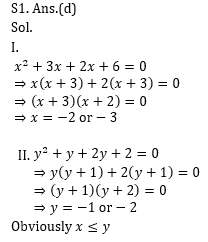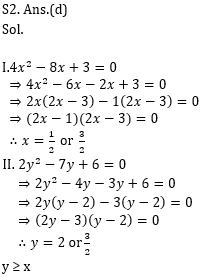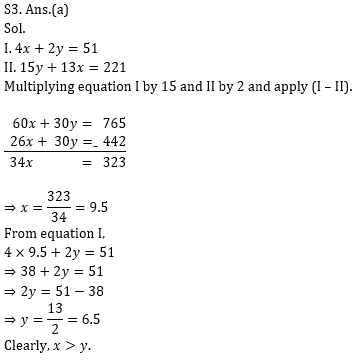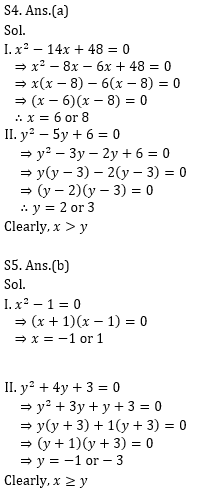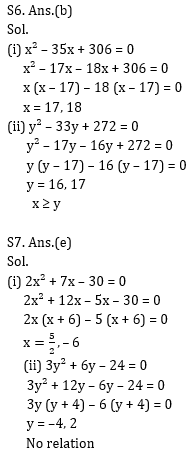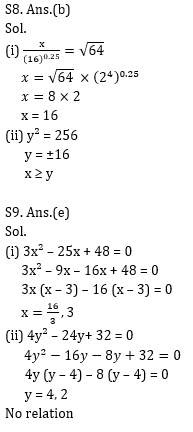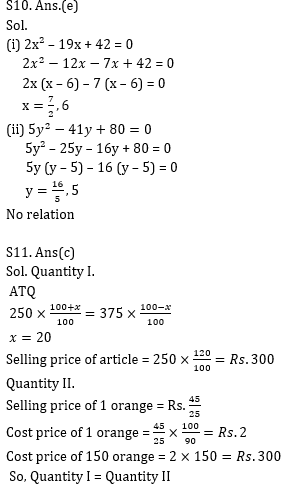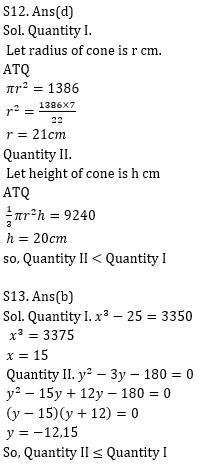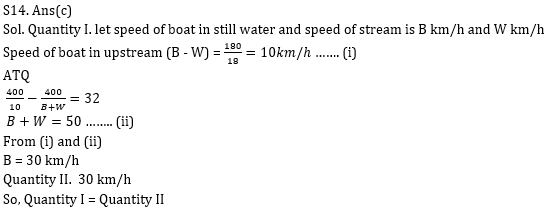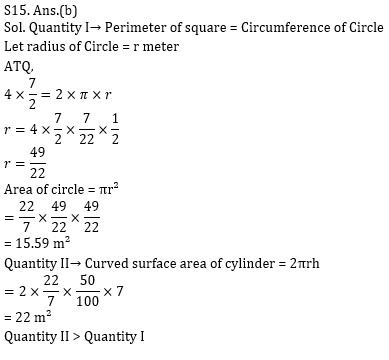Practice with Crash Course and Online Test Series for IBPS Clerk Prelims: